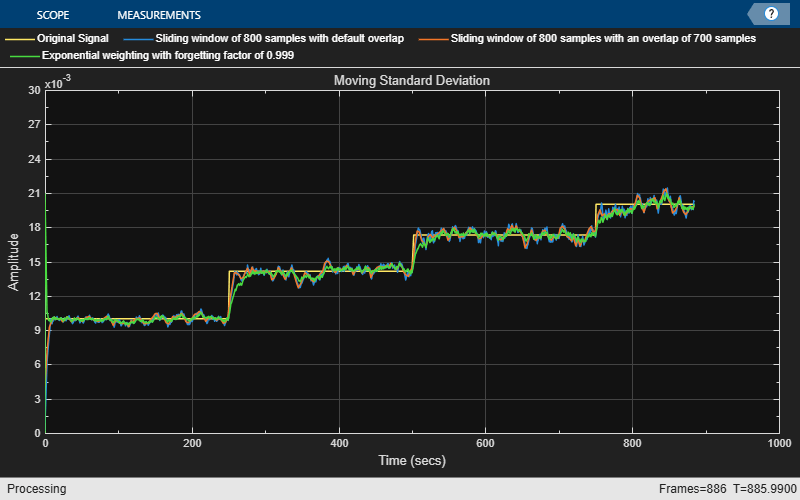# dsp.MovingStandardDeviation

Moving standard deviation

## Description

The `dsp.MovingStandardDeviation` System object™ computes the moving standard deviation of the input signal along each channel, independently over time. The object uses either the sliding window method or the exponential weighting method to compute the moving standard deviation. In the sliding window method, a window of specified length is moved over the data, sample by sample, and the object computes the standard deviation over the data in the window. In the exponential weighting method, the object computes the exponentially weighted moving variance, and takes the square root. For more details on these methods, see Algorithms.

To compute the moving standard deviation of the input:

1. Create the `dsp.MovingStandardDeviation` object and set its properties.

2. Call the object with arguments, as if it were a function.

## Creation

### Syntax

``MovStd = dsp.MovingStandardDeviation``
``MovStd = dsp.MovingStandardDeviation(Len)``
``MovStd = dsp.MovingStandardDeviation(Name,Value)``

### Description

````MovStd = dsp.MovingStandardDeviation` returns a moving standard deviation object, `MovStd`, using the default properties.```

example

````MovStd = dsp.MovingStandardDeviation(Len)` sets the `WindowLength` property to `Len`.```

example

````MovStd = dsp.MovingStandardDeviation(Name,Value)` specifies additional properties using `Name,Value` pairs. Unspecified properties have default values.Example: ```MovStd = dsp.MovingStandardDeviation('Method','Exponential weighting','ForgettingFactor',0.999);``````

## Properties

expand all

Unless otherwise indicated, properties are nontunable, which means you cannot change their values after calling the object. Objects lock when you call them, and the `release` function unlocks them.

If a property is tunable, you can change its value at any time.

• `'Sliding window'` — A window of length specified by `SpecifyWindowLength` is moved over the input data along each channel. For every sample the window moves by, the object computes the standard deviation over the data in the window.

• `'Exponential weighting'` — The object computes the exponentially weighted moving variance, and takes the square root.

For more details on these methods, see Algorithms.

Flag to specify a window length, specified as a scalar boolean.

• `true` — The length of the sliding window is equal to the value you specify in the `WindowLength` property.

• `false` — The length of the sliding window is infinite. In this mode, the standard deviation is computed using the current sample and all the past samples.

#### Dependencies

This property applies when you set `Method` to ```'Sliding window'```.

Length of the sliding window in samples, specified as a positive scalar integer.

#### Dependencies

This property applies when you set `Method` to `'Sliding window'` and `SpecifyWindowLength` to `true`.

Data Types: `single` | `double` | `int8` | `int16` | `int32` | `int64` | `uint8` | `uint16` | `uint32` | `uint64`

Exponential weighting factor, specified as a positive real scalar in the range (0,1].

Since this property is tunable, you can change its value even when the object is locked.

Tunable: Yes

#### Dependencies

This property applies when you set `Method` to `'Exponential weighting'`.

Data Types: `single` | `double`

## Usage

### Syntax

``y = movStd(x)``

### Description

example

````y = movStd(x)` computes the moving standard deviation of the input signal, `x`, using either the sliding window method or exponential weighting method.```

### Input Arguments

expand all

Data input, specified as a vector or a matrix. If `x` is a matrix, each column is treated as an independent channel. The moving standard deviation is computed along each channel. The object also accepts variable-size inputs. Once the object is locked, you can change the size of each input channel, but you cannot change the number of channels.

Data Types: `single` | `double`
Complex Number Support: Yes

### Output Arguments

expand all

Moving standard deviation of the input signal, returned as a vector or a matrix.

Data Types: `single` | `double`
Complex Number Support: Yes

## Object Functions

To use an object function, specify the System object as the first input argument. For example, to release system resources of a System object named `obj`, use this syntax:

`release(obj)`

expand all

 `step` Run System object algorithm `release` Release resources and allow changes to System object property values and input characteristics `reset` Reset internal states of System object

## Examples

collapse all

Compute the moving standard deviation of a noisy square wave signal with varying amplitude using the `dsp.MovingStandardDeviation` object.

Initialization

Set up `movstdWindow` and `movstdExp` objects. `movstdWindow` uses the sliding window method with a window length of 800. `movstdExp` uses the exponential weighting method with a forgetting factor of 0.999. Create a time scope for viewing the output.

```FrameLength = 100; Fs = 100; movstdWindow = dsp.MovingStandardDeviation(800); movstdExp = dsp.MovingStandardDeviation(... 'Method','Exponential weighting',... 'ForgettingFactor',0.999); scope = timescope('SampleRate',Fs,... 'TimeSpanOverrunAction','Scroll',... 'TimeSpanSource','Property',... 'TimeSpan',1000,... 'ShowGrid',true,... 'BufferLength',1e7,... 'YLimits',[0 3e-2]); title = 'Actual (yellow) Sliding Window (blue) Exponentially Weighted (red) standard deviation'; scope.Title = title; ```

Compute the Standard Deviation

Generate a noisy square wave signal. Vary the amplitude of the square wave after a given number of frames. Apply the sliding window method and the exponential weighting method on this signal. The actual standard deviation is `sqrt(np)`. This value is used while adding noise to the data. Compare the actual standard deviation with the computed standard deviation on the time scope.

```count = 1; noisepower = 1e-4 * [1 2 3 4]; for index = 1:length(noisepower) np = noisepower(index); yexp = sqrt(np)*ones(FrameLength,1); for i = 1:250 x = sqrt(np) * randn(FrameLength,1); y1 = movstdWindow(x); y2 = movstdExp(x); scope([yexp,y1,y2]); end end ```## Algorithms

expand all

 Bodenham, Dean. “Adaptive Filtering and Change Detection for Streaming Data.” PH.D. Thesis. Imperial College, London, 2012.

Watch now